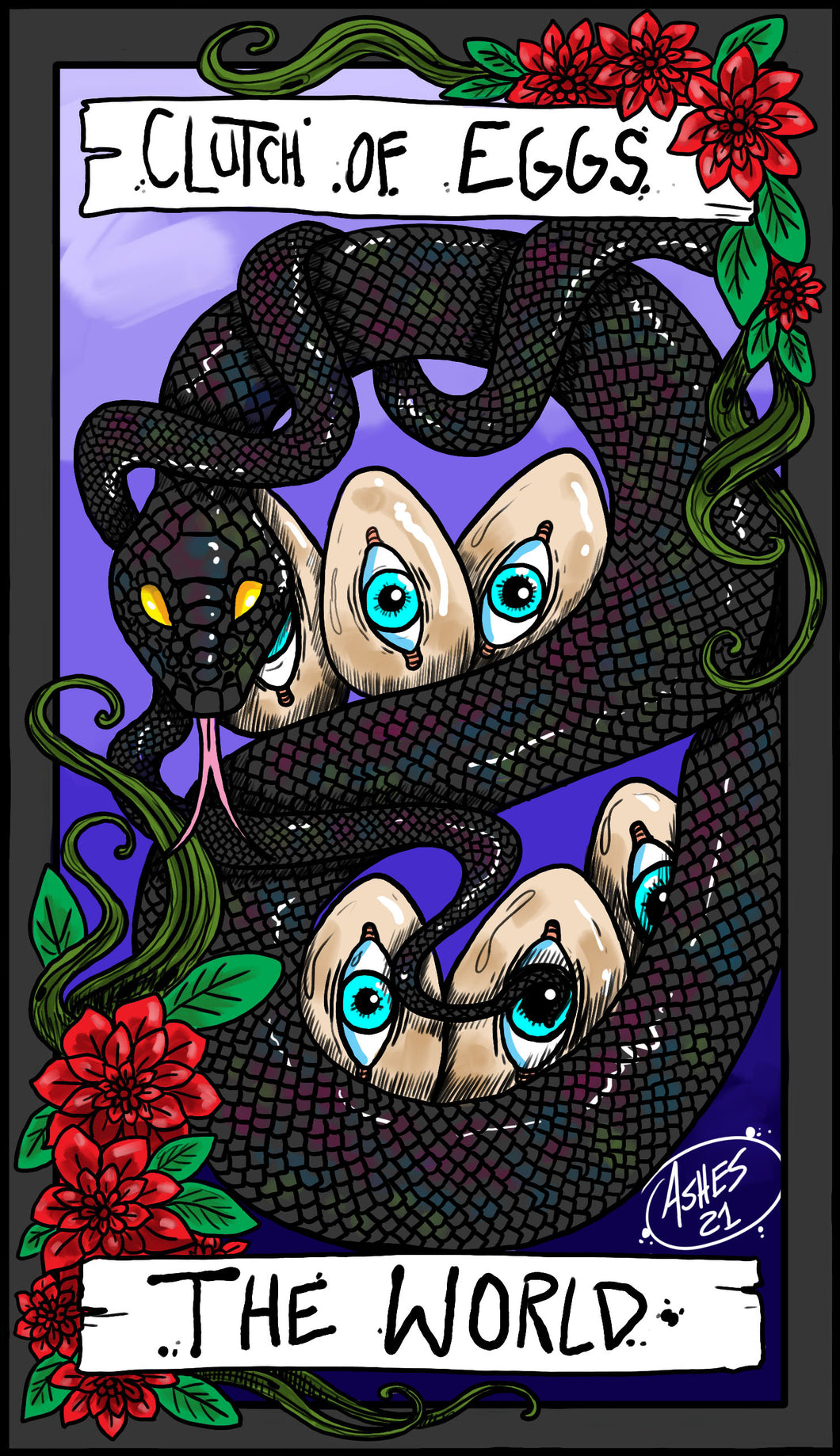# Eggs

The Mermaid Egg Size Large Dimensions:

4 inch total length

5.5 inch circumference at the tip

8.8 inch circumference at the widest point

__________

The Dragon Egg Size Large Dimensions:

4.5 inch total length

6.5 inch circumference at the tip

8.5 inch circumference at the widest point

__________

The Chicken Foot Egg Size Large Dimensions:

4.5 inch total length

6.5 inch circumference at the tip

9 inch circumference at the widest point

__________

The Chicken Foot Egg Regular Size Dimensions:

3 inches total length

5 inch circumference at the tip

7 inch circumference at the widest point

__________

The Mermaid Egg Regular Size Dimensions:

3 inch total length

4 inch circumference at the tip

6.5 inch circumference at the widest point

__________

The Dragon Egg Regular Size Clutch:

3 inches total length

5 inch circumference at the tip

6.4 inch circumference at the widest point

__________

The Mermaid Egg: SIZE MINI DIMENSIONS

Circumference of widest point: 4.5 inches

Total length: 2 inches

__________

The Chicken foot Egg: SIZE MINI DIMENSIONS

circumference of widest point: 4.5 inches

Total length: 2 inches

__________

The Dragon Egg: SIZE MINI DIMENSIONS

Circumference if widest point: 4 inches

Total length: 2 inches

__________

The Alligator Egg, Many Eyed Creature Egg, Winged Treasure, Tentacle Tangle, Beast Seed and The Bat regular size:

Circumference: 5 inches

Length: 2 inches

__________

Toothed Egg Dimensions:

Circumference: 5 1/2 inches

Length: 2 inches

__________

The Alligator Egg Size Large Dimensions:

Circumference at Tip: 6 ½ inches

Circumference at widest point: 7 ½ inches

Total Length: 3 inches

__________

The Tentacle Tangle Size Large Dimensions:

Circumference at tip: 6 ½ inches

Circumference at widest point: 7 ½ inches

Total length: 3 inches

__________

Beast Seed Size Large Dimensions:

Circumference at Tip: 6 inches

Circumference at widest point: 7 ½ inches

Total length: 3 inches

__________

The Toothed One Size Large Dimensions:

Circumference at tip: 7 inches

Circumference at widest point: 8.5 inches

Total Length: 3 inches

__________

The Winged Treasure Size Large Dimensions

Circumference at tip: 6 ½ inches

Circumference at widest point: 7 ½ inches

Total Length: 3 inches

__________

The Bat Egg Size Large Dimensions:

Circumference at Tip: 6 ½ inches

Circumference at widest point: 7 ½ inches

Total Length: 3 inches

__________

The Many Eyed Creature Egg Size Large Dimensions:

Circumference at Tip: 6 ½ inches

Circumference at widest point: 7 ½ inches

Total Length: 3 inches